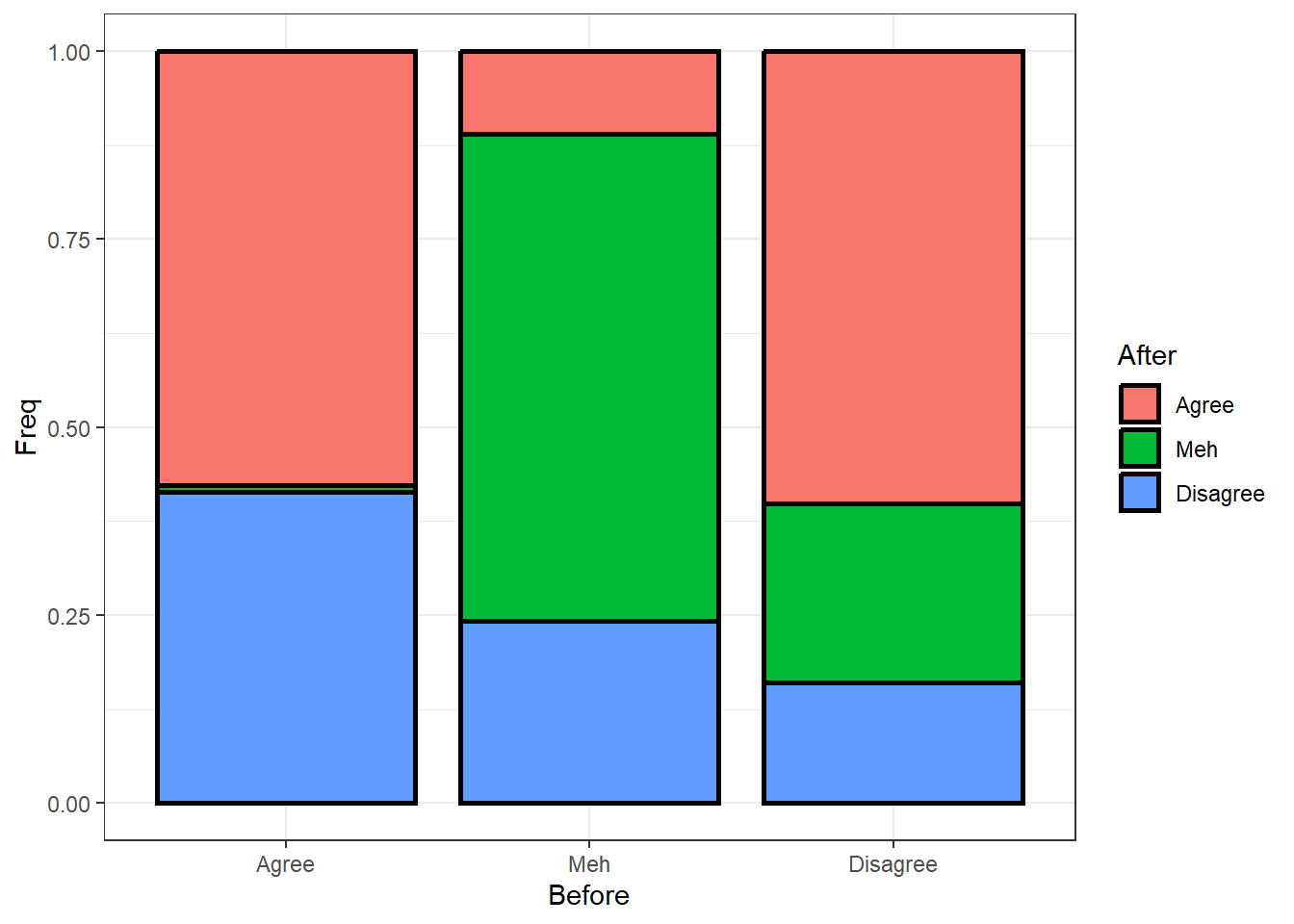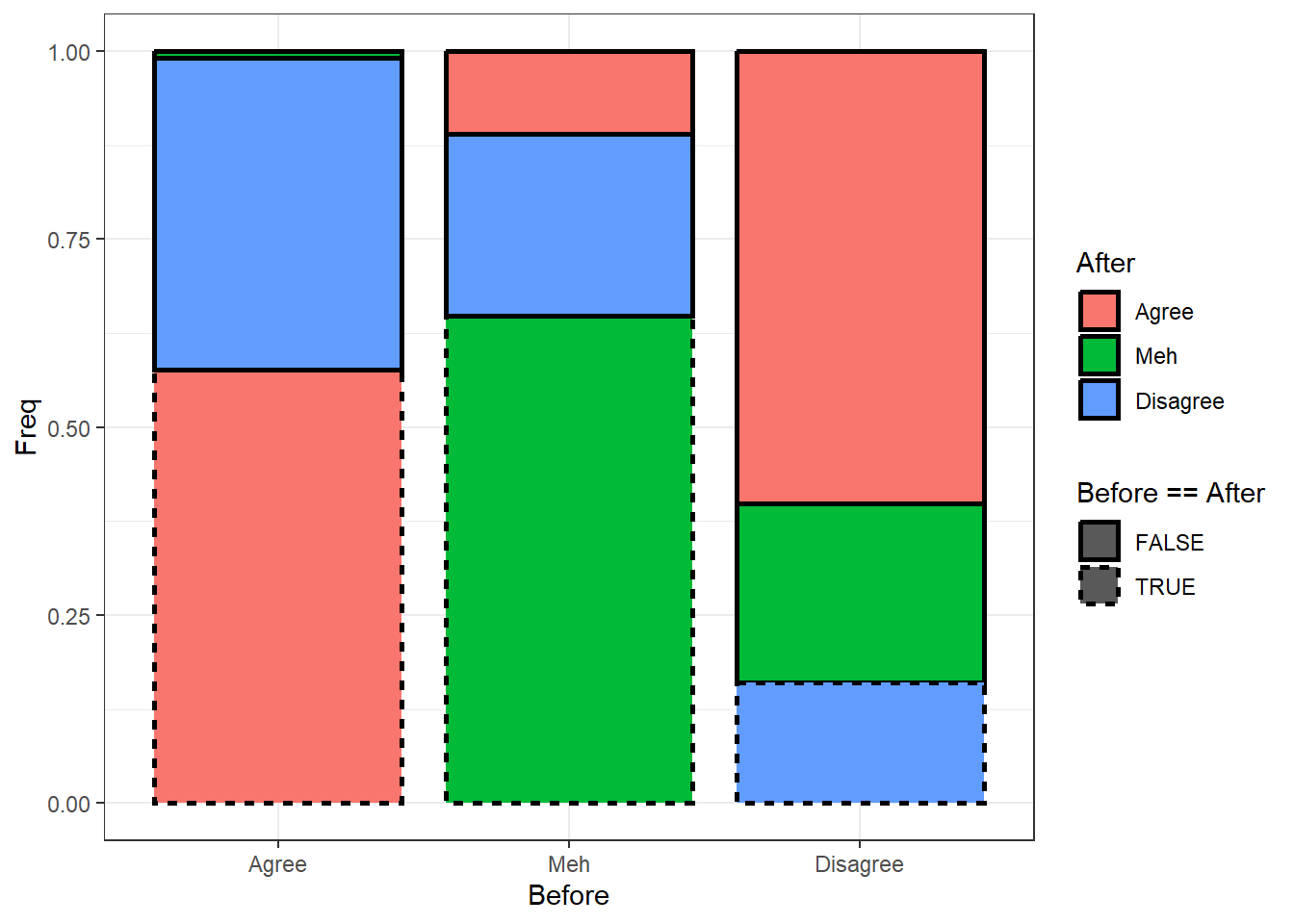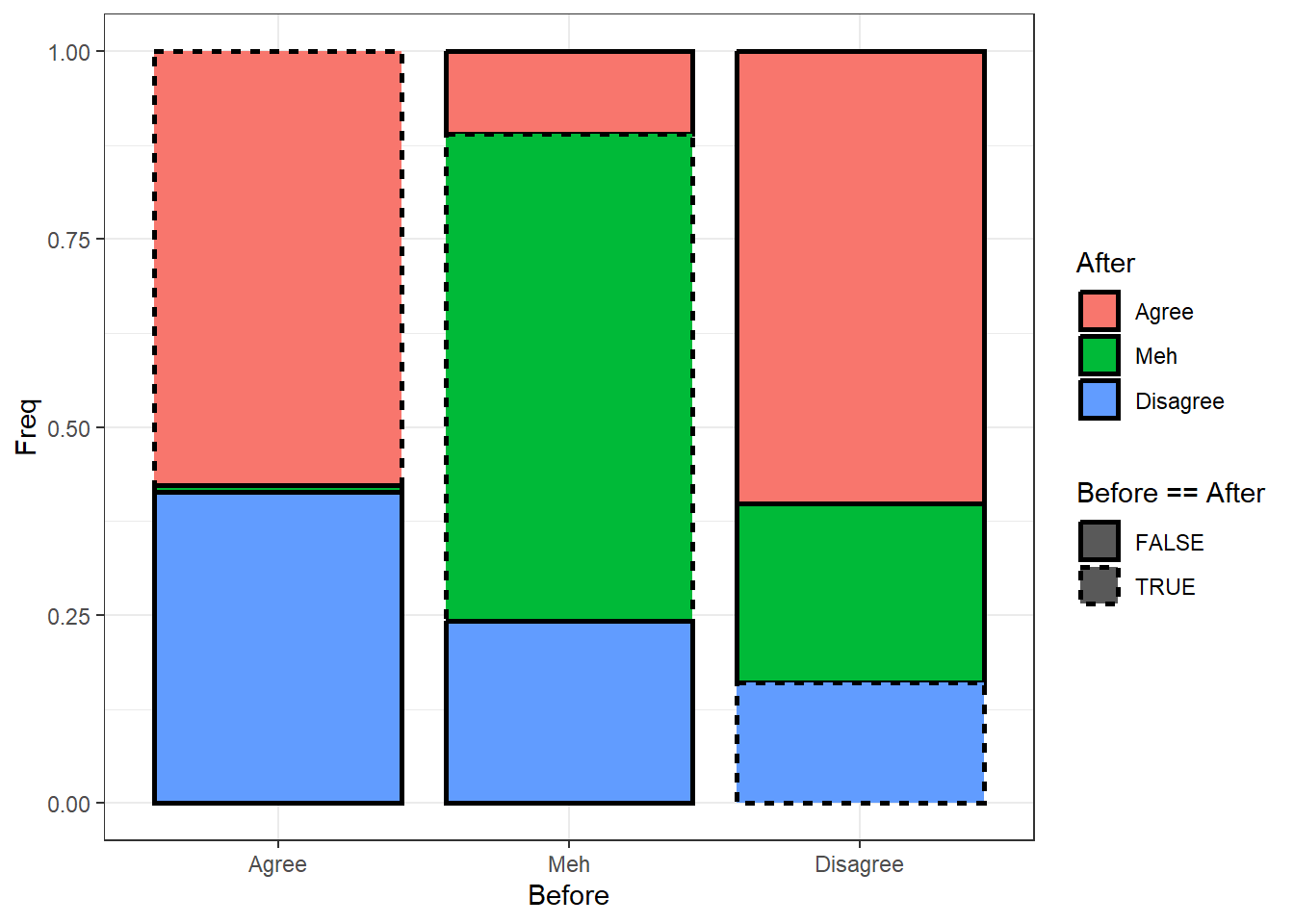# ggplot: the placing and order of aesthetics matters

R
ggplot2
Author

Mattan S. Ben-Shachar

Published

May 16, 2021

A group of people were asked to what degree they agree or disagree with a statement at two time points.

``````Agreement <- matrix(c(794, 150, 86,
12, 888, 34,
570, 333, 23), nrow = 3,
dimnames = list(Before = c("Agree", "Meh", "Disagree"),
After = c("Agree", "Meh", "Disagree")))``````

Our question is how many people changed their minds. Statistically we might use `mcnemar.test()` and `effectsize::cohens_g()`, but we will be focusing on visualization of the data with `ggplot2`.

We first need to re-structure this matrix into a data frame:

``(Agreement_df <- as.data.frame(as.table(Agreement)))``
``````#>     Before    After Freq
#> 1    Agree    Agree  794
#> 2      Meh    Agree  150
#> 3 Disagree    Agree   86
#> 4    Agree      Meh   12
#> 5      Meh      Meh  888
#> 6 Disagree      Meh   34
#> 7    Agree Disagree  570
#> 8      Meh Disagree  333
#> 9 Disagree Disagree   23``````

The basic plot is:

``````library(ggplot2)
theme_set(theme_bw())

ggplot(Agreement_df, aes(Before, Freq, fill = After)) +
geom_col(
position = "fill", width = 0.85,
color = "black", linewidth = 1
)``````Simple enough.

What we want to do is mark the cells where people did not change their response - where `Before` is equal to `After` - with a different line type. We can do this by adding `linetype = Before == After` into the plots aesthetics. This should give diagonal cells a different line-type compared to the other cells. Simple enough, no?

``````ggplot(Agreement_df, aes(Before, Freq, fill = After)) +
geom_col(
position = "fill", width = 0.85,
color = "black", linewidth = 1,
mapping = aes(linetype = Before == After) #<<<<<<<<<
)``````What the hell happened?? The order of cells has changed!

# Grouping & Order of Mapping

The first thing to understand is that we have some implicit grouping going on.

The group aesthetic is by default set to the interaction of all discrete variables in the plot. […] For most applications the grouping is set implicitly by mapping one or more discrete variables to `x`, `y`, `colour`, `fill`, `alpha`, `shape`, `size`, and/or `linetype`.

From the `ggplot2` manual on Aesthetics: grouping

This means that our mapping of `fill` and `linetype` has been used to set the `group`ing of the cells.

The second thing to understand is the order in which these `group`ing aesthetics are used for grouping:

• First, the layer-specific aesthetics are used (in our case, `linetype = Before == After`, which is in the `geom_col()` layer).
• Then (if `inherit.aes = TRUE`, which is the default) any global aesthetics are used (`fill = After`, which is set in the call to `ggplot()`).

This is why the order of the cells has changed: Cells were grouped first by the before-after equality, and only then by the type of “after” response.

# The Fix

The fix is easy, we have to make sure the grouping aesthetics are specified in a way that `ggplot` pulls them in the correct order; that is first by “after” and then by the before-after equality.

Here are all the ways to do that:

## Option 1: Be Explicit

We can explicitly set the `group` aesthetic, using the `interaction()` function, but to add insult to injury, this function must be supplied with the grouping variables in the reverse order (unless you set `lex.order = TRUE`):

``````ggplot(Agreement_df, aes(Before, Freq, fill = After)) +
geom_col(
position = "fill", width = 0.85,
color = "black", linewidth = 1,
mapping = aes(linetype = Before == After,
group = interaction(Before == After, After)) #<<<<<<<<<
)````````````ggplot(Agreement_df, aes(Before, Freq, fill = After)) +
geom_col(
position = "fill", width = 0.85,
color = "black", linewidth = 1,
mapping = aes(linetype = Before == After,
group = interaction(After, Before == After,  #<<<<<<<<<
lex.order = TRUE))       #<<<<<<<<<
) ``````## Option 2: Set All Grouping Aesthetics Globally / By Layer

We can also keep using the implicit setting for the grouping, but set all of the relevant aesthetics globally:

``````# Set both in the global aesthetics:
ggplot(Agreement_df, aes(Before, Freq,
fill = After, linetype = Before == After)) +
geom_col(
position = "fill", width = 0.85,
color = "black", linewidth = 1
)``````Or in the layer itself:

``````# Set both in the layer aesthetics:
ggplot(Agreement_df, aes(Before, Freq)) +
geom_col(
position = "fill", width = 0.85,
color = "black", linewidth = 1,
mapping = aes(fill = After, linetype = Before == After)
)``````Note then even when setting them globally or in the layer, the order still matters:

``````ggplot(Agreement_df, aes(Before, Freq)) +
geom_col(
position = "fill", width = 0.85,
color = "black", linewidth = 1,
mapping = aes(linetype = Before == After, fill = After) # Wrong order
)``````# Conclusion

The location (global or by layer) and order of aesthetics matters. I didn’t know this, and I felt like I was losing my mind; I hope that by writing this post I will be able to spare you some precious keyboard banging and yelps of sorrow.

Code away!